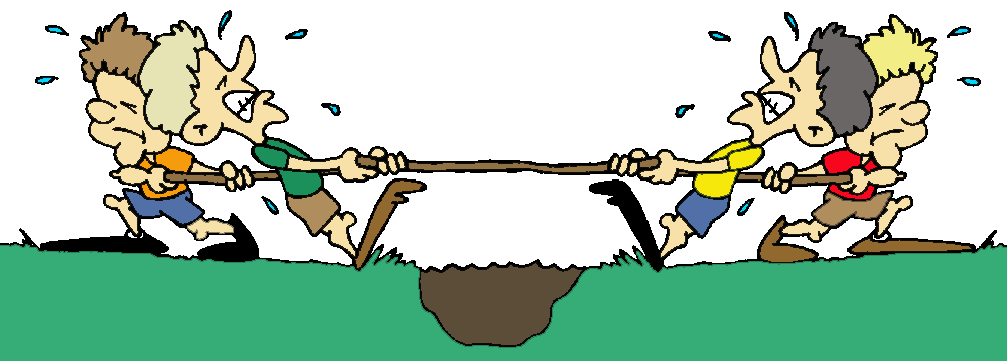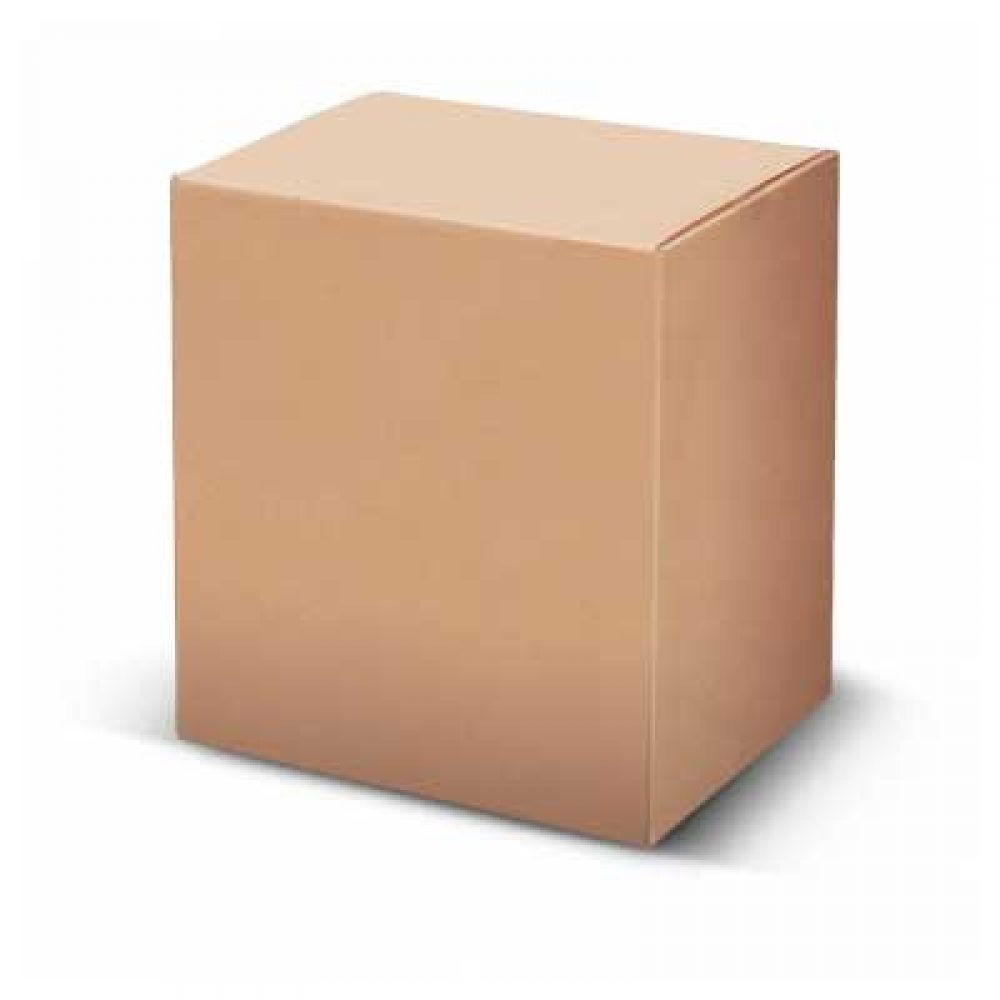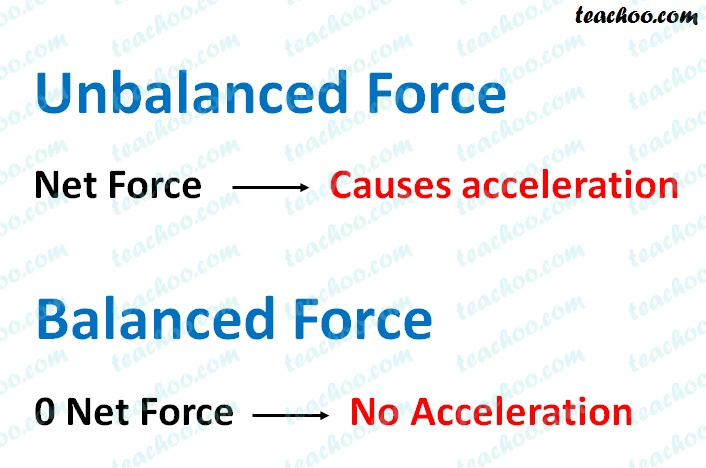Concepts

Class 9
Chapter 9 Class 9 - Force and Laws Of Motion

## What are Balanced Forces?

If resultant total of all forces acting on an object is 0, it is called balanced force

Example

A tug of war game in which both teams exert equal force is example of Balanced Force

A stationary object is an example of Balanced force

Explanation

#### Tug of War Game-Both teams exert Equal ForceIn a tug of war game, 2 teams pull rope from opposite direction

If both teams apply equal strength, the rope does not move

This is because force of 2 teams is balanced (one team exert force from left to right and other team exerts equal force from right to left)

#### Stationary Object is an Example of Balanced force

Suppose a heavy box is kept on ground

It does not move by itself and is stationary

It is because, force of gravity is exerted on box from top to bottom

Also force of reaction is exerted by ground from bottom to top

These both forces balance each other due to which box does not moveNote

Balanced Forces can change shape and size of Object

Example we take inflated balloon and we squeeze it from both sides

It changes shape of balloon

Since we exert force from both sides, it is a balanced force

Hence,we can say that balanced force can change shape and size of object

## What are Unbalanced Force?

If resultant total of all forces acting on an object is not 0, it is called unbalanced force

Example

#### Force Required to Move Stationary Object

Suppose a heavy box is kept on ground

We try to push it with our hands

In this case, if we push it with less force,it does not move (as our muscular force exerted by body is balanced by force of friction)

However, if we push it with more force than the frictional force, it moves because force now is unbalanced force

(as muscular force exerted by our body is more than opposing force of friction)

#### Force Required to Stop Moving Object

A moving ball stops by itself after some time

it is because force of friction exerts opposite force on object

This force of friction is more than force of ball (force is unbalanced) which brings moving ball to rest## Summary and Difference between Balanced and Unbalanced force

 Balanced Force Unbalanced force If resultant total of all forces acting on an object is 0, it is called balanced force If resultant total of all forces acting on an object is not 0, it is called unbalanced force Example Example Force present in Stationary Object Force Required to Move Stationary Object Force present in Object moving with constant velocity Force Required to Stop Moving Object Tug of War Game-Both teams exert Equal Force Tug of War Game-Both teams exert Equal Force This force cannot move stationary object This force can move stationary object This force cannot stop moving object This force can stop moving object

## Questions

NCERT Question 1 -  An object experiences a net zero external unbalanced force. Is it possible for the object to be travelling with a non-zero velocity? If yes, state the conditions that must be placed on the magnitude and direction of the velocity. If no, provide a reason.

Learn in your speed, with individual attention - Teachoo Maths 1-on-1 Class